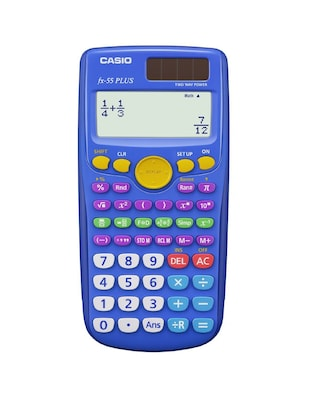Can you use an extra \$850 annually? Become a member today.
Close

# Casio® FX-55PLUS Fraction Scientific Calculator Teacher Pack, 10/Pack

Item #: 901-895862DHT
Model #: FX55PLUSTP
Brand: Casio\$179.99 Each
Out of Stock
This item is currently unavailable.
Notify me when this product is back in stock
Please note: Pricing is subject to change.
Here are some other options to think about...
Description
Casio® Fraction scientific calculator having 12 digit dot matrix display allows to perform basic calculations such as addition, subtraction, multiplication, conversion operation and is sold as 10 per pack. Casio? Engineering/scientific calculator helps students to learn elementary mathematics division with remainder and random numbers. Calculator helps you to solve complex problems, logarithmic functions, HMS conversion.
• Display type: Dot Matrix
• Display screen: 12 digit - Dot Matrix
• Power source: Battery
• Memory: M+, M-, MR (RCL M), MC / Store M (STO M), x-M -, Variables(x, Y...) M, Answer memory
• Basic calculations: +, -, *, /, division with remainder, +/- -, (-), ( ), factorial, pi, x-y
• HMS unit, HMS, HMS conversion
• Fraction calculations: b/c, a*b/c, a*b/c (-) b/c, Simplify, F(-)D
• Percent: Percent calculation, percent conversion
• Logarithmic functions: log, logab, ln, 10^x, e^x
• Powers and roots: x^2, x^3, x^y, 1/x, x^(1/2), x^(1/3), x^(1/y)
• Intuitive functions
• Fraction simplification
• Easy fraction/decimal conversion
• Includes 10 scientific calculators, instruction manual
Specifications
 Brand : Casio Bulk Item : Yes Color Family : Black Customizable : No Depth in Inches : 2.20 Features : Scrolling Keys,Backspace Height in Inches : 16.00 Number of Digits Displayed : 12+ Number of Lines : 2 Power Source : Battery Solar Powered : No True Color : Black Used For : Indoor and Outdoor Weight : 4.85 lbs. per Each Width in Inches : 13.80 Weight : 4.85 lbs. per Each

### AccessoriesLoading...